#### 期刊菜单

Stability Analysis of Cohen-Grossberg Neural Networks with Proportional Delays

Abstract: This paper investigates global exponential stability of Cohen-Grossberg neural networks with proportional delays. Firstly, the Cohen-Grossberg neural networks model with proportional delay is equivalent to the Cohen-Grossberg neural networks model with constant delay through appropriate transformation. Sufficient conditions for global exponential stability are established by applying M-matrix theory and inequality techniques. The validity of the obtained conclusions is verified by numerical simulation.

1. 引言

Cohen-Grossberg神经网络  (Cohen-Grossberg neural networks, CGNNs)是一种特殊的递归神经网络。CGNNs比较普遍，涵盖了细胞神经网络  和Hopfield神经网络  。递归神经网络在图像处理    、优化   ，模式识别   ，联想记忆和并行计算等领域得到了广泛的应用。

2. 数学模型及预备知识

$\left\{\begin{array}{l}{\stackrel{˙}{u}}_{i}\left(t\right)=-{a}_{i}\left({u}_{i}\left(t\right)\right)\left[{b}_{i}\left({u}_{i}\left(t\right)\right)-\underset{j=1}{\overset{n}{\sum }}\text{ }\text{ }{c}_{ij}{f}_{j}\left({u}_{j}\left(t\right)\right)-\underset{j=1}{\overset{n}{\sum }}\text{ }\text{ }{d}_{ij}{g}_{j}\left({u}_{j}\left({q}_{j}t\right)\right)+{I}_{i}\right],\text{\hspace{0.17em}}\text{\hspace{0.17em}}t\ge 1\\ {u}_{i}\left(s\right)={\phi }_{i}\left(s\right),\text{\hspace{0.17em}}\text{\hspace{0.17em}}s\in \left[\rho ,1\right],\text{\hspace{0.17em}}\text{\hspace{0.17em}}i=1,2,\cdots ,n\end{array}$ (2.1)

$\left\{\begin{array}{l}{\stackrel{˙}{x}}_{i}\left(t\right)=-{e}^{t}{a}_{i}\left({x}_{i}\left(t\right)\right)\left[{b}_{i}\left({x}_{i}\left(t\right)\right)-\underset{j=1}{\overset{n}{\sum }}\text{ }\text{ }{c}_{ij}{f}_{j}\left({x}_{j}\left(t\right)\right)-\underset{j=1}{\overset{n}{\sum }}\text{ }\text{ }{d}_{ij}{g}_{j}\left({x}_{j}\left(t-{\tau }_{j}\right)\right)+{I}_{i}\right]\\ {x}_{i}\left(s\right)={\psi }_{i}\left(s\right),\text{\hspace{0.17em}}\text{\hspace{0.17em}}s\in \left[-p,0\right],\text{\hspace{0.17em}}\text{\hspace{0.17em}}i=1,2,\cdots ,n\end{array}$ (2.2)

${\alpha }_{i}^{-}\le {a}_{i}\left(u\right)\le {\alpha }_{i}^{+},\text{\hspace{0.17em}}\text{\hspace{0.17em}}\forall u\in R$

$\frac{{b}_{i}\left(u\right)-{b}_{i}\left(v\right)}{u-v}\ge {B}_{i},\text{\hspace{0.17em}}\text{\hspace{0.17em}}\forall u,v\in R\text{ }\text{ }且\text{ }\text{ }u\ne v$

$|{f}_{j}\left(u\right)-{f}_{j}\left(v\right)|\le {F}_{j}|u-v|,\text{\hspace{0.17em}}\text{\hspace{0.17em}}\forall u,v\in R\text{ }\text{ }且\text{ }\text{ }u\ne v$

$|{g}_{j}\left(u\right)-{g}_{j}\left(v\right)|\le {G}_{j}|u-v|,\text{\hspace{0.17em}}\text{\hspace{0.17em}}\forall u,v\in R\text{ }\text{ }且\text{ }\text{ }u\ne v$

$B=diag\left({B}_{1},{B}_{2},\cdots ,{B}_{n}\right),F=diag\left({F}_{1},{F}_{2},\cdots ,{F}_{n}\right),G=diag\left({G}_{1},{G}_{2},\cdots ,{G}_{n}\right)$

1) 矩阵A是非奇异M-矩阵。

2) 存在非零向量 $\xi ={\left({\xi }_{1},{\xi }_{2},\cdots ,{\xi }_{n}\right)}^{\text{T}}$ 使得 $A\xi >0$

$|{x}_{i}\left(t\right)-{x}_{i}^{*}|\le K‖\psi -{x}^{*}‖{\text{e}}^{-\lambda t},t\ge 0,$

$‖\psi -{x}^{*}‖=\underset{s\in \left[-\tau ,0\right]}{\mathrm{sup}}|{\psi }_{i}\left(s\right)-{x}_{i}^{*}|$

3. 主要结论

$\left\{\begin{array}{l}{\stackrel{˙}{y}}_{i}\left(t\right)=-{\text{e}}^{t}{\stackrel{¯}{a}}_{i}\left({y}_{i}\left(t\right)\right)\left[{\stackrel{¯}{b}}_{i}\left({y}_{i}\left(t\right)\right)-\underset{j=1}{\overset{n}{\sum }}\text{ }\text{ }{c}_{ij}{\stackrel{¯}{f}}_{j}\left({y}_{j}\left(t\right)\right)-\underset{j=1}{\overset{n}{\sum }}\text{ }\text{ }{d}_{ij}{\stackrel{¯}{g}}_{j}\left({y}_{j}\left(t-{\tau }_{j}\right)\right)+{I}_{i}\right]\\ {y}_{i}\left(s\right)={\varphi }_{i}\left(s\right),\text{\hspace{0.17em}}\text{\hspace{0.17em}}s\in \left[-p,0\right],\text{\hspace{0.17em}}\text{\hspace{0.17em}}i=1,2,\cdots ,n\end{array}$ (3.1)

${\stackrel{¯}{a}}_{i}\left({y}_{i}\right)={a}_{i}\left({y}_{i}-{x}_{i}^{*}\right)-{a}_{i}\left({x}_{i}^{*}\right),\text{\hspace{0.17em}}\text{\hspace{0.17em}}{\stackrel{¯}{b}}_{i}\left({y}_{i}\right)={b}_{i}\left({y}_{i}-{x}_{i}^{*}\right)-{b}_{i}\left({x}_{i}^{*}\right)$

${\stackrel{¯}{f}}_{j}\left({y}_{j}\right)={f}_{j}\left({y}_{j}-{x}_{j}^{*}\right)-{f}_{j}\left({x}_{j}^{*}\right),\text{\hspace{0.17em}}\text{\hspace{0.17em}}{\stackrel{¯}{g}}_{j}\left({y}_{j}\right)={g}_{j}\left({y}_{j}-{x}_{j}^{*}\right)-{g}_{j}\left({x}_{j}^{*}\right)$

${\varphi }_{i}\left(s\right)={\psi }_{i}\left(s\right)-{x}_{i}^{*},\text{\hspace{0.17em}}\text{\hspace{0.17em}}i,j=1,2,\cdots ,n$

$-{B}_{i}{\xi }_{i}+\underset{j=1}{\overset{N}{\sum }}\text{ }\text{ }{\xi }_{j}|{c}_{ij}|{F}_{j}+\underset{j=1}{\overset{N}{\sum }}\text{ }\text{ }{\xi }_{j}|{d}_{ij}|{G}_{j}<0$ (3.2)

${H}_{i}\left(x\right)=-\left({B}_{i}-{x}_{i}\right){\xi }_{i}+\underset{j=1}{\overset{N}{\sum }}\text{ }\text{ }{\xi }_{j}|{c}_{ij}|{F}_{j}+\underset{j=1}{\overset{N}{\sum }}\text{ }\text{ }{\text{e}}^{{x}_{j}\tau }{\xi }_{j}|{d}_{ij}|{G}_{j}$ (3.3)

${H}_{i}\left(0\right)=-{B}_{i}{\xi }_{i}+\underset{j=1}{\overset{N}{\sum }}\text{ }\text{ }{\xi }_{j}|{c}_{ij}|{F}_{j}+\underset{j=1}{\overset{N}{\sum }}\text{ }\text{ }{\xi }_{j}|{d}_{ij}|{G}_{j}<0$ (3.4)

${H}_{i}\left(\sigma \right)=-\left({B}_{i}-{\sigma }_{i}\right){\xi }_{i}+\underset{j=1}{\overset{N}{\sum }}\text{ }\text{ }{\xi }_{j}|{c}_{ij}|{F}_{j}+\underset{j=1}{\overset{N}{\sum }}\text{ }\text{ }{\text{e}}^{{\sigma }_{j}\tau }{\xi }_{j}|{d}_{ij}|{G}_{j}=0$ (3.5)

${H}_{i}\left({\sigma }^{-}\right)=-\left({B}_{i}-{\sigma }_{i}^{-}\right){\xi }_{i}+\underset{j=1}{\overset{N}{\sum }}\text{ }\text{ }{\xi }_{j}|{c}_{ij}|{F}_{j}+\underset{j=1}{\overset{N}{\sum }}\text{ }\text{ }{\text{e}}^{{\sigma }_{j}^{-}\tau }{\xi }_{j}|{d}_{ij}|{G}_{j}<0$ (3.6)

${V}_{i}\left(t\right)={\text{e}}^{\lambda t}|{y}_{i}\left(t\right)|,i=1,2,\cdots ,n$ (3.7)

${V}_{i}\left(t\right)$ 求右上导：

$\begin{array}{c}{D}^{+}{V}_{i}\left(t\right)={\text{e}}^{\lambda t}\mathrm{sgn}\left({y}_{i}\left(t\right)\right)\left\{-{\text{e}}^{t}{\stackrel{¯}{a}}_{i}\left({y}_{i}\left(t\right)\right)\left[{\stackrel{¯}{b}}_{i}\left({y}_{i}\left(t\right)\right)-\underset{j=1}{\overset{n}{\sum }}\text{ }\text{ }{c}_{ij}{\stackrel{¯}{f}}_{j}\left({y}_{j}\left(t\right)\right)-\underset{j=1}{\overset{n}{\sum }}\text{ }\text{ }{d}_{ij}{\stackrel{¯}{g}}_{j}\left({y}_{j}\left(t-{\tau }_{j}\right)\right)\right]\right\}+\lambda {\text{e}}^{\lambda t}|{y}_{i}\left(t\right)|\\ \le -{\text{e}}^{\lambda t}{\text{e}}^{t}{\stackrel{¯}{a}}_{i}\left({y}_{i}\left(t\right)\right)\left[|{\stackrel{¯}{b}}_{i}\left({y}_{i}\left(t\right)\right)|-|\underset{j=1}{\overset{n}{\sum }}\text{ }\text{ }{c}_{ij}{\stackrel{¯}{f}}_{j}\left({y}_{j}\left(t\right)\right)|-|\underset{j=1}{\overset{n}{\sum }}\text{ }\text{ }{d}_{ij}{\stackrel{¯}{g}}_{j}\left({y}_{j}\left(t-{\tau }_{j}\right)\right)|\right]+\lambda {\text{e}}^{\lambda t}|{y}_{i}\left(t\right)|\end{array}$

$\begin{array}{l}\le -{\text{e}}^{\lambda t}{\text{e}}^{t}{\stackrel{¯}{a}}_{i}\left({y}_{i}\left(t\right)\right)\left[{B}_{i}|\left({y}_{i}\left(t\right)\right)|-\underset{j=1}{\overset{n}{\sum }}|{c}_{ij}|{F}_{j}|{y}_{i}\left(t\right)|-\underset{j=1}{\overset{n}{\sum }}|{d}_{ij}|{G}_{j}|{y}_{j}\left(t-{\tau }_{j}\right)|\right]+\lambda {\text{e}}^{\lambda t}|{y}_{i}\left(t\right)|\\ \le -{\text{e}}^{t}{\stackrel{¯}{a}}_{i}\left({y}_{i}\left(t\right)\right)\left[{B}_{i}{\text{e}}^{\lambda t}|\left({y}_{i}\left(t\right)\right)|-\underset{j=1}{\overset{n}{\sum }}|{c}_{ij}|{F}_{j}{\text{e}}^{\lambda t}|{y}_{i}\left(t\right)|-\underset{j=1}{\overset{n}{\sum }}\text{ }\text{ }{\text{e}}^{\lambda {\tau }_{j}}|{d}_{ij}|{G}_{j}{\text{e}}^{\lambda \left(t-{\tau }_{j}\right)}|{y}_{j}\left(t-{\tau }_{j}\right)|\right]+\lambda {\text{e}}^{\lambda t}|{y}_{i}\left(t\right)|\end{array}$

$\begin{array}{l}\le -{\text{e}}^{t}{\stackrel{¯}{a}}_{i}\left({y}_{i}\left(t\right)\right)\left[{B}_{i}{V}_{i}\left(t\right)-\underset{j=1}{\overset{n}{\sum }}|{c}_{ij}|{F}_{j}{V}_{i}\left(t\right)-\underset{j=1}{\overset{n}{\sum }}\text{ }\text{ }{\text{e}}^{\lambda {\tau }_{j}}|{d}_{ij}|{G}_{j}{V}_{i}\left(t-{\tau }_{j}\right)\right]+\lambda {\text{e}}^{t}{\stackrel{¯}{a}}_{i}\left({y}_{i}\left(t\right)\right){V}_{i}\left(t\right)\\ ={\text{e}}^{t}{\stackrel{¯}{a}}_{i}\left({y}_{i}\left(t\right)\right)\left[-\left({B}_{i}-\lambda \right){V}_{i}\left(t\right)+\underset{j=1}{\overset{n}{\sum }}|{c}_{ij}|{F}_{j}{V}_{i}\left(t\right)+\underset{j=1}{\overset{n}{\sum }}\text{ }\text{ }{\text{e}}^{\lambda {\tau }_{j}}|{d}_{ij}|{G}_{j}{V}_{i}\left(t-{\tau }_{j}\right)\right],\text{\hspace{0.17em}}\text{\hspace{0.17em}}i=1,2,\cdots ,n,\text{\hspace{0.17em}}t\ge 0\end{array}$ (3.8)

$q=\frac{‖\psi -{x}^{*}‖}{{\mathrm{min}}_{1\le i\le n}\left\{{\xi }_{i}\right\}}$ ，则

${V}_{i}\left(s\right)={\text{e}}^{\lambda s}|{y}_{i}\left(s\right)|\le |{\varphi }_{i}\left(s\right)|=|{\psi }_{i}\left(s\right)-{x}_{i}^{*}|\le {\xi }_{i}q$ (3.9)

${V}_{i}\left(t\right)\le {\xi }_{i}q,\text{\hspace{0.17em}}\text{\hspace{0.17em}}t\ge 0,\text{\hspace{0.17em}}i=1,2,\cdots ,n$ (3.10)

${V}_{i}\left({t}^{*}\right)\le {\xi }_{i}q,{D}^{*}\left({V}_{i}\left({t}^{*}\right)\right)\ge 0$ ，且当 $t\in \left[-\rho ,{t}^{*}\right]$ 时， ${V}_{i}\left(t\right)\le {\xi }_{i}q$ (3.11)

$\begin{array}{c}{D}^{+}{V}_{i}\left({t}^{*}\right)\le {\text{e}}^{{t}^{*}}{\stackrel{¯}{a}}_{i}\left({y}_{i}\left({t}^{*}\right)\right)\left[-\left({B}_{i}-\lambda \right){V}_{i}\left({t}^{*}\right)+\underset{j=1}{\overset{n}{\sum }}|{c}_{ij}|{F}_{j}{V}_{i}\left({t}^{*}\right)+\underset{j=1}{\overset{n}{\sum }}\text{ }\text{ }{\text{e}}^{\lambda {\tau }_{j}}|{d}_{ij}|{G}_{j}{V}_{i}\left({t}^{*}-{\tau }_{j}\right)\right]\\ <0\end{array}$ (3.12)

$|{y}_{i}\left(t\right)|\le {\text{e}}^{-\lambda t}{\xi }_{i}q\le {\text{e}}^{-\lambda t}\frac{{\sum }_{i=1}^{n}{\xi }_{i}}{\mathrm{min}\left\{{\xi }_{i}\right\}}‖\psi -{x}^{*}‖=K‖\psi -{x}^{*}‖{\text{e}}^{-\lambda t}$

$|{x}_{i}\left(t\right)-{x}^{*}|\le K‖\psi -{x}^{*}‖{\text{e}}^{-\lambda t}$ (3.13)

4. 数值实验

$\left\{\begin{array}{l}{\stackrel{˙}{u}}_{1}\left(t\right)=-{a}_{1}\left({u}_{1}\left(t\right)\right)\left[{b}_{1}\left({u}_{1}\left(t\right)\right)-\underset{j=1}{\overset{2}{\sum }}\text{ }\text{ }{c}_{1j}{f}_{j}\left({u}_{j}\left(t\right)\right)-\underset{j=1}{\overset{2}{\sum }}\text{ }\text{ }{d}_{1j}{g}_{j}\left({u}_{j}\left(0.6t\right)\right)\right]\\ {\stackrel{˙}{u}}_{2}\left(t\right)=-{a}_{2}\left({u}_{2}\left(t\right)\right)\left[{b}_{2}\left({u}_{2}\left(t\right)\right)-\underset{j=1}{\overset{2}{\sum }}\text{ }\text{ }{c}_{2j}{f}_{j}\left({u}_{j}\left(t\right)\right)-\underset{j=1}{\overset{2}{\sum }}\text{ }\text{ }{d}_{2j}{g}_{j}\left({u}_{j}\left(0.8t\right)\right)\right]\end{array}$ (4.1)

${a}_{1}\left(u\right)=1+0.5\mathrm{cos}\left(u\right),\text{\hspace{0.17em}}{a}_{2}\left(u\right)=2+0.5\mathrm{cos}\left(u\right);\text{\hspace{0.17em}}{b}_{1}\left(u\right)=5u,\text{\hspace{0.17em}}{b}_{2}\left(u\right)=12u$

${f}_{i}\left(u\right)=0.5\left(|u+1|-|u-1|\right),\text{\hspace{0.17em}}{g}_{i}\left(u\right)=\mathrm{tanh}\left(u\right),\text{\hspace{0.17em}}i=1,2$

$C={\left({c}_{ij}\right)}_{2×2}=\left[\begin{array}{cc}1& -2\\ 2& 1\end{array}\right],\text{\hspace{0.17em}}D={\left({d}_{ij}\right)}_{2×2}=\left[\begin{array}{cc}1& -1\\ -1& 1\end{array}\right]$

${\alpha }_{1}^{-}=0.5,\text{\hspace{0.17em}}{\alpha }_{1}^{+}=1.5,\text{\hspace{0.17em}}{\alpha }_{2}^{-}=1.5,\text{\hspace{0.17em}}{\alpha }_{2}^{+}=2.5$

$B=diag\left(5,12\right),F=G=diag\left(1,1\right)$

$B-|C|F-|D|G=\left[\begin{array}{cc}3& -3\\ -3& 10\end{array}\right]$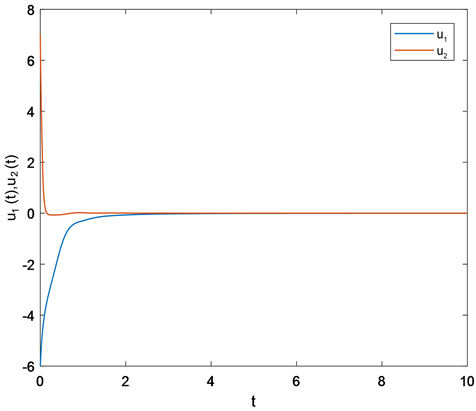Figure 1. State response of (4.1)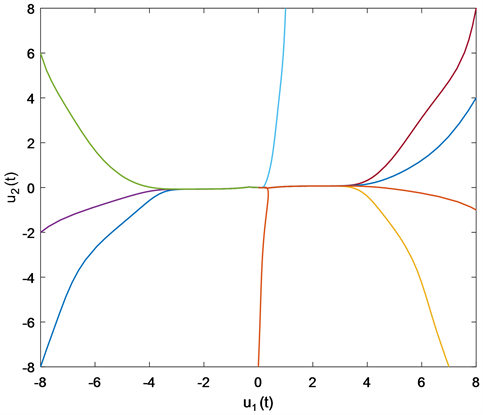Figure 2. Phase trajectories of system (4.1)

$\left\{\begin{array}{l}{\stackrel{˙}{u}}_{1}\left(t\right)=-{a}_{1}\left({u}_{1}\left(t\right)\right)\left[{b}_{1}\left({u}_{1}\left(t\right)\right)-\underset{j=1}{\overset{2}{\sum }}\text{ }\text{ }{c}_{1j}{f}_{j}\left({u}_{j}\left(t\right)\right)-\underset{j=1}{\overset{2}{\sum }}\text{ }\text{ }{d}_{1j}{g}_{j}\left({u}_{j}\left(0.5t\right)\right)-1\right]\\ {\stackrel{˙}{u}}_{2}\left(t\right)=-{a}_{2}\left({u}_{2}\left(t\right)\right)\left[{b}_{2}\left({u}_{2}\left(t\right)\right)-\underset{j=1}{\overset{2}{\sum }}\text{ }\text{ }{c}_{2j}{f}_{j}\left({u}_{j}\left(t\right)\right)-\underset{j=1}{\overset{2}{\sum }}\text{ }\text{ }{d}_{2j}{g}_{j}\left({u}_{j}\left(0.5t\right)\right)+1\right]\end{array}$ (4.2)

${a}_{1}\left(u\right)=1+0.5\mathrm{cos}\left(u\right),\text{\hspace{0.17em}}{a}_{2}\left(u\right)=2+0.5\mathrm{cos}\left(u\right);\text{\hspace{0.17em}}{b}_{1}\left(u\right)=5u,\text{\hspace{0.17em}}{b}_{2}\left(u\right)=12u$

${f}_{i}\left(u\right)=0.5\left(|u+1|-|u-1|\right),\text{\hspace{0.17em}}{g}_{i}\left(u\right)=\mathrm{tanh}\left(u\right),\text{\hspace{0.17em}}i=1,2$

$C={\left({c}_{ij}\right)}_{2×2}=\left[\begin{array}{cc}1& -2\\ 2& 1\end{array}\right],\text{\hspace{0.17em}}D={\left({d}_{ij}\right)}_{2×2}=\left[\begin{array}{cc}1& -1\\ -1& 1\end{array}\right]$

${\alpha }_{1}^{-}=0.5,\text{\hspace{0.17em}}{\alpha }_{1}^{+}=1.5,\text{\hspace{0.17em}}{\alpha }_{2}^{-}=1.5,\text{\hspace{0.17em}}{\alpha }_{2}^{+}=2.5$

$B=diag\left(5,12\right),F=G=diag\left(1,1\right)$

$B-|C|F-|D|G=\left[\begin{array}{cc}3& -3\\ -3& 10\end{array}\right]$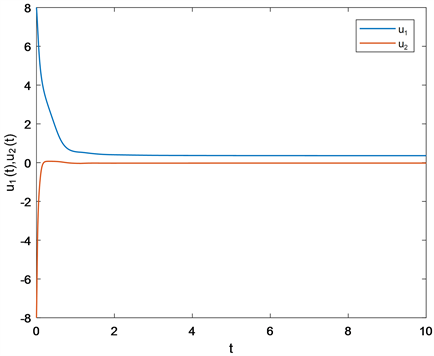Figure 3. State response of (4.2)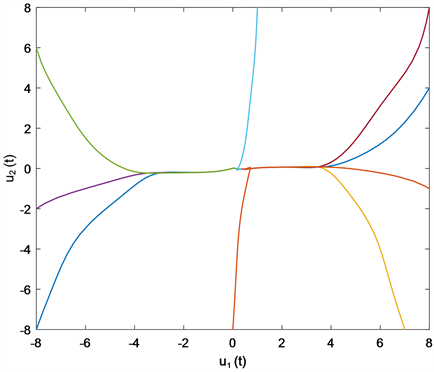Figure 4. Phase trajectories of system (4.2)

$\left\{\begin{array}{l}{\stackrel{˙}{u}}_{1}\left(t\right)=-{a}_{1}\left({u}_{1}\left(t\right)\right)\left[{b}_{1}\left({u}_{1}\left(t\right)\right)-\underset{j=1}{\overset{3}{\sum }}\text{ }\text{ }{c}_{1j}{f}_{j}\left({u}_{j}\left(t\right)\right)-\underset{j=1}{\overset{3}{\sum }}\text{ }\text{ }{d}_{1j}{g}_{j}\left({u}_{j}\left(0.5t\right)\right)\right]\\ {\stackrel{˙}{u}}_{2}\left(t\right)=-{a}_{2}\left({u}_{2}\left(t\right)\right)\left[{b}_{2}\left({u}_{2}\left(t\right)\right)-\underset{j=1}{\overset{3}{\sum }}\text{ }\text{ }{c}_{1j}{f}_{j}\left({u}_{j}\left(t\right)\right)-\underset{j=1}{\overset{3}{\sum }}\text{ }\text{ }{d}_{1j}{g}_{j}\left({u}_{j}\left(0.6t\right)\right)\right]\\ {\stackrel{˙}{u}}_{3}\left(t\right)=-{a}_{3}\left({u}_{3}\left(t\right)\right)\left[{b}_{3}\left({u}_{3}\left(t\right)\right)-\underset{j=1}{\overset{3}{\sum }}\text{ }\text{ }{c}_{1j}{f}_{j}\left({u}_{j}\left(t\right)\right)-\underset{j=1}{\overset{3}{\sum }}\text{ }\text{ }{d}_{1j}{g}_{j}\left({u}_{j}\left(0.8t\right)\right)\right]\end{array}$ (4.3)

${a}_{1}\left(u\right)=1+0.5\mathrm{cos}\left(u\right),\text{\hspace{0.17em}}{a}_{2}\left(u\right)=1+0.5\mathrm{cos}\left(u\right),\text{\hspace{0.17em}}{a}_{3}\left(u\right)=2+0.5\mathrm{sin}\left( u \right)$

${b}_{1}\left(u\right)=6u,\text{\hspace{0.17em}}{b}_{2}\left(u\right)=8u,\text{\hspace{0.17em}}{b}_{3}\left(u\right)=10u$

${f}_{i}\left(u\right)=0.5\left(|u+1|-|u-1|\right),\text{\hspace{0.17em}}{g}_{i}\left(u\right)=\mathrm{tanh}\left(u\right),\text{\hspace{0.17em}}i=1,2,3$

$C={\left({c}_{ij}\right)}_{3×3}=\left[\begin{array}{ccc}1& -2& 2\\ 2& -1& -1\\ -1& 3& 2\end{array}\right],\text{\hspace{0.17em}}\text{\hspace{0.17em}}D={\left({d}_{ij}\right)}_{3×3}=\left[\begin{array}{ccc}1& -1& 2\\ -1& -1& -2\\ 2& -1& -1\end{array}\right]$

${\alpha }_{1}^{-}={\alpha }_{2}^{-}=0.5,\text{\hspace{0.17em}}{\alpha }_{3}^{-}=1.5;\text{\hspace{0.17em}}\text{\hspace{0.17em}}{\alpha }_{1}^{+}={\alpha }_{2}^{+}=1.5,\text{\hspace{0.17em}}{\alpha }_{3}^{+}=2.5$

$B=diag\left(5,8,10\right),F=G=diag\left(1,1,1\right)$

$B-|C|F-|D|G=\left[\begin{array}{ccc}4& -3& -4\\ -3& 7& -3\\ -3& -4& 7\end{array}\right]$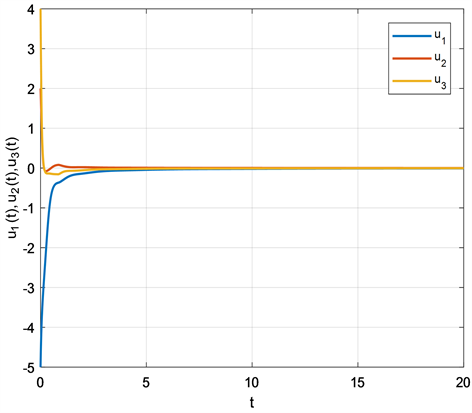Figure 5. State response of (4.3)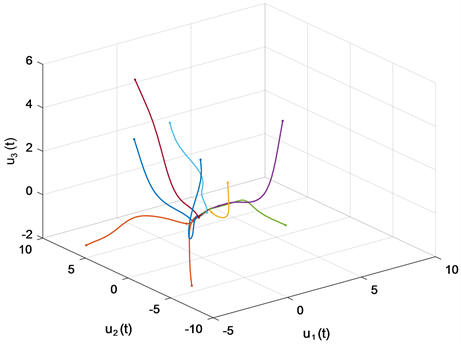Figure 6. Phase trajectories of system (4.3)

5. 总结

NOTES

*通讯作者。

  Cohen, M.A. and Grossberg, S. (1983) Absolute Stability of Global Pattern Formation and Parallel Memory Storage by Competitive Neural Networks. IEEE Transactions on Systems, Man, and Cybernetics, SMC-13, 815-826. https://doi.org/10.1109/TSMC.1983.6313075  Chua, L.O. and Yang, L. (1988) Cellular Neural Networks: Theory. IEEE Transactions on Circuits and Systems, 35, 1257-1272. https://doi.org/10.1109/31.7600  Hopfield, J.J. (1982) Neural Networks and Physical Systems with Emergent Collective Computational Abilities. Proceedings of the National Academy of Sciences, 79, 2554-2558. https://doi.org/10.1073/pnas.79.8.2554  Hu, X., Feng, G. and Duan, S. (2015) Multilayer RTD-Memristor-Based Cellular Neural Networks for Color Image Processing. Neurocomputing, 162, 150-162. https://doi.org/10.1016/j.neucom.2015.03.057  Hu, X., Feng, G. and Duan, S. (2017) A Memristive Multilayer Cellular Neural Network with Applications to Image Processing. IEEE Transactions on Neural Networks and Learning Systems, 28, 1889-1901. https://doi.org/10.1109/TNNLS.2016.2552640  Li, J. and Peng, Z. (2015) Multi-Source Image Fusion Algo-rithm Based on Cellular Neural Networks with Genetic Algorithm. Optik, 126, 5230-5236. https://doi.org/10.1016/j.ijleo.2015.09.187  Nagamani, G. and Radhika, T. (2016) A Quadratic Convex Com-bination Approach on Robust Dissipativity and Passivity Analysis for Takagi-Sugeno Fuzzy Cohen-Grossberg Neural Networks with Time-Varying Delays. Mathematical Methods in the Applied Sciences, 39, 3880-3896. https://doi.org/10.1002/mma.3835  Hayakawa, Y. and Nakajima, K. (2010) Design of the Inverse Function Delayed Neural Network for Solving Combinatorial Optimization Problems. IEEE Transactions on Neural Networks, 21, 224-237. https://doi.org/10.1109/TNN.2009.2035618  Velichko, A., Belyaev, M. and Boriskov, P. (2019) A Model of an Oscillatory Neural Network with Multilevel Neurons for Pattern Recognition and Computing. Electronics, 8, 75. https://doi.org/10.3390/electronics8010075  Zhang, Y., Li, Y. and Wang, X. (2017) Synaptic Characteristics of Ag/AgInSbTe/Ta-Based Memristor for Pattern Recognition Applications. IEEE Transactions on Electron Devices, 64, 1806-1811. https://doi.org/10.1109/TED.2017.2671433  Baldi, P. and Atiya, A.F. (1994) How Delays Affect Neural Dy-namics and Learning. IEEE Transactions on Neural Networks, 5, 612-621. https://doi.org/10.1109/72.298231  Faydasicok, O. (2020) New Criteria for Global Stability of Neutral-Type Cohen-Grossberg Neural Networks with Multiple Delays. Neural Networks, 125, 330-337. https://doi.org/10.1016/j.neunet.2020.02.020  Zhu, H.Y., Zhu, Q.X., Sun, X.B. and Zhou, H.W. (2016) Ex-istence and Exponential Stability of Pseudo Almost Automorphic Solutions for Cohen-Grossberg Neural Networks with Mixed Delays. Advances in Continuous and Discrete Models, 2016, Article No. 120. https://advancesindifferenceequations.springeropen.com/articles/10.1186/s13662-016-0831-5  Zhao, W. (2008) Global Exponential Stability Analysis of Cohen-Grossberg Neural Network with Delays. Communications in Nonlinear Science and Numerical Simulation, 13, 847-856. https://doi.org/10.1016/j.cnsns.2006.09.004  Aouiti, C. and Dridi, F. (2019) New Results on Impulsive Cohen-Grossberg Neural Networks. Neural Processing Letters, 49, 1459-1483. https://doi.org/10.1007/s11063-018-9880-y  Lu, D.J. and Li, C.J. (2013) Exponential Stability of Stochastic High-Order BAM Neural Networks with Time Delays and Impulsive Effects. Neural Computing and Applications, 23, 1-8. https://link.springer.com/article/10.1007/s00521-012-0861-1  Zeng, H.-B., Liu, X.-G. and Wang, W. (2019) A Generalized Free-Matrix-Based Integral Inequality for Stability Analysis of Time-Varying Delay Systems. Applied Mathematics and Computation, 354, 1-8. https://doi.org/10.1016/j.amc.2019.02.009  Zhang, C.-K., He, Y. and Jiang, L. (2016) Stability Analysis of Systems with Time-Varying Delay via Relaxed Integral Inequalities. Systems & Control Letters, 92, 52-61. https://doi.org/10.1016/j.sysconle.2016.03.002  Li, Z., Yan, H. and Zhang, H. (2020) Stability Analysis of Linear Systems with Time-Varying Delay via Intermediate Polynomial-Based Functions. Automatica, 113, Article ID: 108756. https://doi.org/10.1016/j.automatica.2019.108756  Bazighifan, O., Elabbasy, E.M. and Moaaz, O. (2019) Oscillation of Higher-Order Differential Equations with Distributed Delay. Journal of Inequalities and Applica-tions, 2019, 55. https://doi.org/10.1186/s13660-019-2003-0  Cao, Y. (2019) Bifurcations in an Internet Con-gestion Control System with Distributed Delay. Applied Mathematics and Computation, 347, 54-63. https://doi.org/10.1016/j.amc.2018.10.093  Zhao, Y., Li, X. and Cao, J. (2020) Global Exponential Stability for Impulsive Systems with Infinite Distributed Delay Based on Flexible Impulse Frequency. Applied Mathematics and Computation, 386, Article ID: 125467. https://doi.org/10.1016/j.amc.2020.125467  Zhou, L.Q. (2013) Delay-Dependent Exponential Stability of Cellular Neural Networks with Multi-Proportional Delays. Neural Processing Letters, 38, 347-359. https://link.springer.com/article/10.1007/s11063-012-9271-8  Zhou, L. (2014) Global Asymptotic Stability of Cellular Neural Networks with Proportional Delays. Nonlinear Dynamics, 77, 41-47. https://doi.org/10.1007/s11071-014-1271-y  Zhou, L. and Zhao, Z. (2022) Global Polynomial Periodicity and Polynomial Stability of Proportional Delay Cohen-Grossberg Neural Networks. ISA Transactions, 122, 205-217. https://doi.org/10.1016/j.isatra.2021.04.041  Shen, W., Zhang, X. and Wang, Y. (2020) Stability Analysis of High Order Neural Networks with Proportional Delays. Neurocomputing, 372, 33-39. https://doi.org/10.1016/j.neucom.2019.09.019  Yang, Z., Zhang, J. and Hu, J. (2021) New Results on Fi-nite-Time Stability for Fractional-Order Neural Networks with Proportional Delay. Neurocomputing, 442, 327-336. https://doi.org/10.1016/j.neucom.2021.02.082  Zhou, L. (2013) Delay-Dependent Exponential Stability of Cellular Neural Networks with Multi-Proportional Delays. Neural Processing Letters, 38, 347-359. https://doi.org/10.1007/s11063-012-9271-8  Cao, J. and Wang, J. (2003) Global Asymptotic Stability of a General Class of Recurrent Neural Networks with Time-Varying Delays. IEEE Transactions on Circuits and Systems I: Fundamental Theory and Applications, 50, 34-44. https://doi.org/10.1109/TCSI.2002.807494  Zhang, J. (2003) Globally Exponential Stability of Neural Net-works with Variable Delays. IEEE Transactions on Circuits and Systems I: Fundamental Theory and Applications, 50, 288-290. https://doi.org/10.1109/TCSI.2002.808208